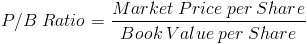# Price to Book ValueThe Price to Book Ratio formula, sometimes referred to as the market to book ratio, is used to compare a company's net assets available to common shareholders relative to the sale price of its stock. The formula for price to book value is the stock price per share divided by the book value per share.

The stock price per share can be found as the amount listed as such through the secondary stock market.

The book value per share is considered to be the total equity for common stockholders which can be found on a company's balance sheet.

## Use of Price to Book Value Formula

The price to book value formula can be used by investors to show how the market perceives the value of a particular stock to be. A ratio over one implies that the market is willing to pay more than the equity per share. A ratio under one implies that the market is willing to pay less.

A price to book value of less than one can imply that the company is not running up to par. This, along with other factors, could also lead to a hostile takeover.

## Issues with the Price to Book Value Formula

One may argue that a ratio under one implies that the company is perceived as being a worse investment than if it were above one. On the other hand, another may argue that the stock is underpriced and a favorable investment. Due to these discrepancies of opinion, using other stock valuation methods along with or apart from the price to book value formula may be beneficial.

Another issue with the price to book value formula is that there are many underlying factors that can affect the formula such as issuing new stock, paying dividends, and stock repurchases. It is possible for a company to manipulate this ratio by various means which is why it is important to not use any one particular financial formula in isolation.

New to Finance?